The slab of a material of refractive index 2 shown in figure has curved surface APB of radius of curvature 10 cm and a plane surface CD. On the left of APB is air and on the right of CD is water with refractive indices as given in figure. An object O is placed at a distance of 15 cm from pole P as shown. The distance of the final image of O from P, as viewed from the left is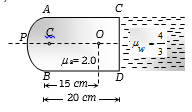(a)   20 cm

(b)   30 cm

(c)   40 cm

(d)   50 cm

Concept Questions :-

Lenses
High Yielding Test Series + Question Bank - NEET 2020

Difficulty Level:

The distance between a convex lens and a plane mirror is 10 cm. The parallel rays incident on the convex lens after reflection from the mirror form image at the optical centre of the lens. Focal length of the lens will be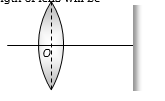(a)   10 cm

(b)   20 cm

(c)   30 cm

(d)   Cannot be determined

Concept Questions :-

Lenses
High Yielding Test Series + Question Bank - NEET 2020

Difficulty Level:

An air bubble in a sphere having 4 cm diameter that appears 1 cm from the surface nearest to the eye when looked along diameter. If${}_{a}\mu _{g}$ = 1.5, the distance of bubble from the refracting surface is

(a) 1.2 cm                    (b) 3.2 cm

(c) 2.8 cm                    (d) 1.6 cm

Concept Questions :-

Lenses
High Yielding Test Series + Question Bank - NEET 2020

Difficulty Level:

An observer can see through a pinhole the top end of a thin rod of height h, placed as shown in the figure. The beaker height is 3h and its radius h. When the beaker is filled with a liquid up to a height 2h, he can see the lower end of the rod. Then the refractive index of the liquid is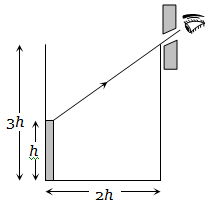(a) $5/2$                                    (b) $\sqrt{\left(5/2\right)}$

(c) $\sqrt{\left(3/2\right)}$                               (d) 3/2

Concept Questions :-

Refraction at plane surface
High Yielding Test Series + Question Bank - NEET 2020

Difficulty Level:

In an experiment of find the focal length of a concave mirror a graph is drawn between the magnitudes of u and v.  The graph looks like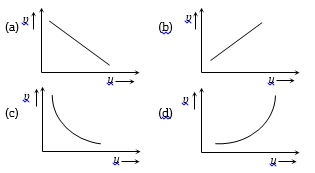Concept Questions :-

Reflection at spherical surface
High Yielding Test Series + Question Bank - NEET 2020

Difficulty Level:

As the position of an object (u) reflected from a concave mirror is varied, the position of the image (v) also varies. By letting the u change from 0 to infinity, the graph between v versus u will be-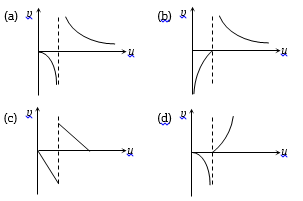Concept Questions :-

Reflection at spherical surface
High Yielding Test Series + Question Bank - NEET 2020

Difficulty Level:

The graph between u and v for a convex mirror is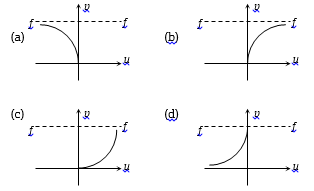Concept Questions :-

Reflection at spherical surface
High Yielding Test Series + Question Bank - NEET 2020

Difficulty Level:

A glass prism $\left(\mu =1.5\right)$ is dipped in water $\left(\mu =4/3\right)$ as shown in figure. A light ray is incident normally on the surface AB. It reaches the surface BC after totally reflected, if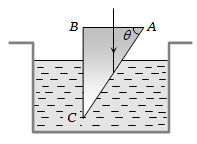(a)                             (b)

(c)                             (d) It is not possible

Concept Questions :-

Total internal reflection
High Yielding Test Series + Question Bank - NEET 2020

Difficulty Level:

A convex lens A of focal length 20 cm and a concave lens B of focal length 5 cm are kept along the same axis with the distance d between them. If a parallel beam of light falling on A leaves B as a parallel beam, then distance d in cm will be

(a) 25                         (b) 15

(c) 30                          (d) 50

Concept Questions :-

Lenses
High Yielding Test Series + Question Bank - NEET 2020

Difficulty Level:

A medium shows relation between i and r as shown. If speed of light in the medium is nc then value of n is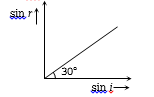(a)   1.5

(b)   2

(c)   2–1

(d)   3–1/2

Concept Questions :-

Refraction at plane surface